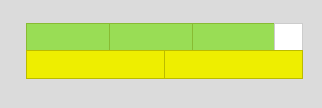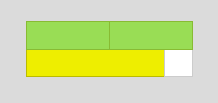#### You may also like### N000ughty Thoughts

How many noughts are at the end of these giant numbers?### DOTS Division

Take any pair of two digit numbers x=ab and y=cd where, without loss of generality, ab > cd . Form two 4 digit numbers r=abcd and s=cdab and calculate: {r^2 - s^2} /{x^2 - y^2}.### Mod 3

Prove that if a^2+b^2 is a multiple of 3 then both a and b are multiples of 3.

# Different by One

##### Age 14 to 16Challenge Level
If you have not used the Cuisenaire Environment before, you might find it helpful to look at the instructions and video before trying this problem.

Choose two different colours of rod, and make a line of each colour.
Can you make your two lines differ by the length of one white rod?

For example, here are three greens and two yellows. The length of the greens is one less than the length of the yellows.”‹
They could also be arranged like this:This time, the length of the greens is one more than the length of the yellows.

Try some different pairs of rods and look for arrangements where the lengths differ by one.

Here are some questions you might like to explore:

If you start with two rods that differ in length by 2, when is it possible to make two lines that differ by 1?
How many of each rod will you need?

What if you could use rods longer than 10, for example 23 and 25? Or 99 and 101? Or...

Once you've found an arrangement where the two lines differ by one, is there a quick way to find other arrangements with the same colour rods that also differ by one?

What if you start with two rods that differ in length by 3? By 4?